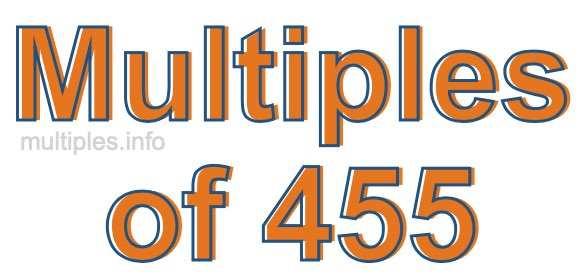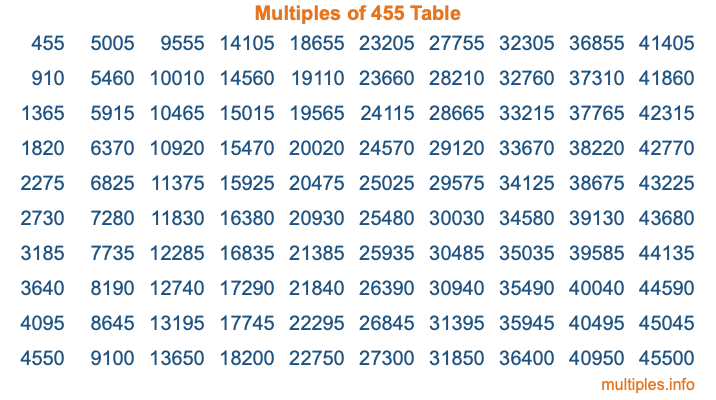Multiples of 455Welcome to the Multiples of 455 page. Here we will first teach you everything you will ever need to know about the multiples of 455, and then give you a study guide summary of everything we taught you to make sure you remember it all. Use this page to look up facts and learn information about the multiples of 455. This page will make you a multiples of four hundred fifty-five expert!

Definition of Multiples of 455
Multiples of 455 are all the numbers that when divided by 455 equal an integer. Each of the multiples of 455 are called a multiple. A multiple of 455 is created by multiplying 455 by an integer.

Therefore, to create a list of multiples of 455, you start with 1 multiplied by 455, then 2 multiplied by 455, then 3 multiplied by 455, and so on for as long as you want. Thus, the list of the first five multiples of 455 is 455, 910, 1365, 1820, and 2275. To see a larger list of multiples of 455, see the printable image of Multiples of 455 further down on this page. We also have a category where you can choose any nth multiple of 455.

Multiples of 455 Checker
The Multiples of 455 Checker below checks to see if any number of your choice is a multiple of 455. In other words, it checks to see if there is any number (integer) that when multiplied by 455 will equal your number. To do that, we divide your number by 455. If the the quotient is an integer, then your number is a multiple of 455.

Is  a multiple of 455?

Least Common Multiple of 455 and ...
A Least Common Multiple (LCM) is the lowest multiple that two or more numbers have in common. This is also called the smallest common multiple or lowest common multiple and is useful to know when you are adding our subtracting fractions. Enter one or more numbers below (455 is already entered) to find the LCM.

Check out our LCM Calculator if you need more details about the Least Common Multiple or if you need the LCM for different numbers for adding and subtraction fractions.

nth Multiple of 455
As we stated above, 455 is the first multiple of 455, 910 is the second multiple of 455, 1365 is the third multiple of 455, and so on. Enter a number below to find the nth multiple of 455.

th multiple of 455

Multiples of 455 vs Factors of 455
455 is a multiple of 455 and a factor of 455, but that is where the similarities end. All postive multiples of 455 are 455 or greater than 455. All positive factors of 455 are 455 or less than 455.

Below is the beginning list of multiples of 455 and the factors of 455 so you can compare:

Multiples of 455: 455, 910, 1365, 1820, 2275, etc.

Factors of 455: 1, 5, 7, 13, 35, 65, 91, 455

As you can see, the multiples of 455 are all the numbers that you can divide by 455 to get a whole number. The factors of 455, on the other hand, are all the whole numbers that you can multiply by another whole number to get 455.

It's also interesting to note that if a number (x) is a factor of 455, then 455 will also be a multiple of that number (x).

Multiples of 455 vs Divisors of 455
The divisors of 455 are all the integers that 455 can be divided by evenly. Below is a list of the divisors of 455.

Divisors of 455: 1, 5, 7, 13, 35, 65, 91, 455

The interesting thing to note here is that if you take any multiple of 455 and divide it by a divisor of 455, you will see that the quotient is an integer.

Multiples of 455 Table
Below is an image of the first 100 multiples of 455 in a table. The table is in chronological order, column by column. The first column has the first ten multiples of 455, the second column has the next ten multiples of 455, and so on.The Multiples of 455 Table is also referred to as the 455 Times Table or Times Table of 455. You are welcome to print out our table for your studies.

Negative Multiples of 455
Although not often discussed or needed in math, it is worth mentioning that you can make a list of negative multiples of 455 by multiplying 455 by -1, then by -2, then by -3, and so on, to get the following list of negative multiples of 455:

-455, -910, -1365, -1820, -2275, etc.

Multiples of 455 Summary
Below is a summary of important Multiples of 455 facts that we have discussed on this page. To retain the knowledge on this page, we recommend that you read through the summary and explain to yourself or a study partner why they hold true.

There are an infinite number of multiples of 455.

A multiple of 455 divided by 455 will equal a whole number.

455 divided by a factor of 455 equals a divisor of 455.

The nth multiple of 455 is n times 455.

The largest factor of 455 is equal to the first positive multiple of 455.

455 is a multiple of every factor of 455.

455 is a multiple of 455.

A multiple of 455 divided by a divisor of 455 equals an integer.

455 divided by a divisor of 455 equals a factor of 455.

Any integer times 455 will equal a multiple of 455.

Multiples of a Number
Here you can get the multiples of another number, all with the same attention to detail as we did for multiples of 455 on this page.

Multiples of
Multiples of 456
Did you find our page about multiples of four hundred fifty-five educational? Do you want more knowledge? Check out the multiples of the next number on our list!